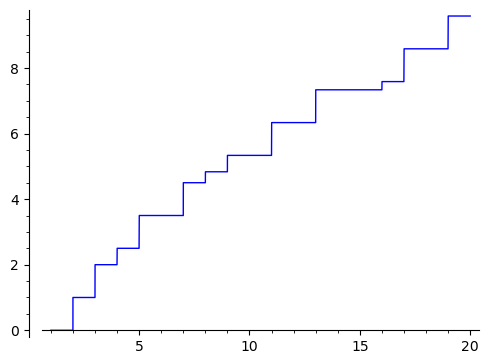# Revision history [back]

Many such plotting problems are solved by using lambda functions.

In this case:

p = plot(lambda x: J(x), (1, 20))
p


Many such plotting problems are solved by using using a callable function or a lambda functions.function instead of a symbolic expression.

In this case:The problem is that J(x) tries to evaluate J with the symbolic variable x as an argument, and it cannot make log(x)/log(2) an integer while x stays symbolic.

To work around that, plot using J rather than J(x):

p = plot(J, (1, 20))
p


or use a lambda function:

p = plot(lambda x: J(x), (1, 20))
p


Many such plotting problems are solved by using a callable function or a lambda function instead of a symbolic expression.

The problem is that J(x) tries to evaluate J with the symbolic variable x as an argument, and it cannot make log(x)/log(2) an integer while x stays symbolic.

To work around that, plot using J rather than J(x):

p = plot(J, (1, 20))
p


or use a lambda function:

p = plot(lambda x: J(x), (1, 20))
pThe function J could be rewritten as:

def J(x):
return sum(1/i * prime_pi(x**(1/i))
for i in range(1, int(log(x)/log(2)) + 1))